# AP Board 4th Class Maths Solutions 9th Lesson Fractions

Andhra Pradesh AP Board 4th Class Maths Solutions 9th Lesson Fractions Textbook Exercise Questions and Answers.

## AP State Syllabus 4th Class Maths Solutions Chapter 9 Fractions

In the following picture pupils are participating in a painting competition. Can you identify pupils who have completed half ?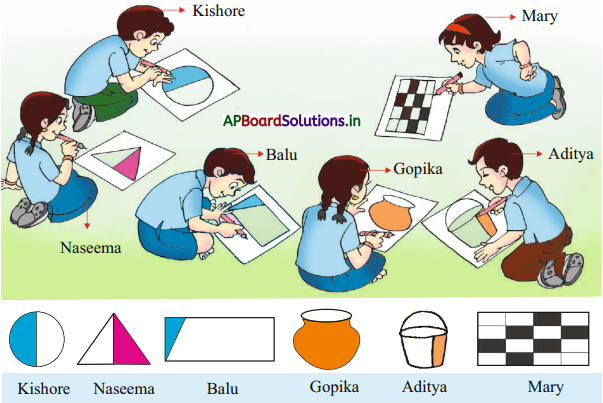a) Kishore has done half of the picture. (True/False)
Solution:
True

b) Aditya has done completely. (True/False)
Solution:
False

c) Balu has done half of the picture. (True/False)
Solution:
Falsed) Gopika has completed the picture. (True/False)
Solution:
True

e) Mary has done half of the table. (True/False)
Solution:
False

f) Naseema has done half of the triangle. (True/False)
Solution:
True

Question 1.
Write the numbers that denote the apples in the box.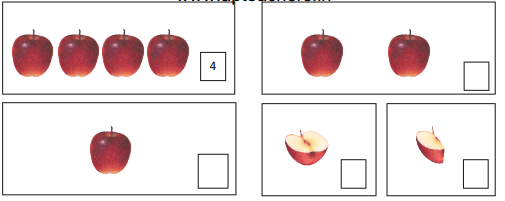Solution: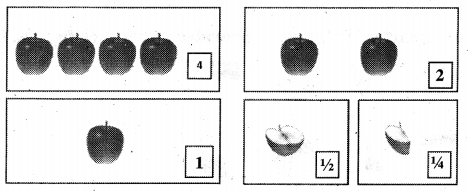Question 2.
In the following, some pictures are shown as a whole and some in halves. Write “W for whole, ‘H’ for the half and ‘Q’ for the quarter.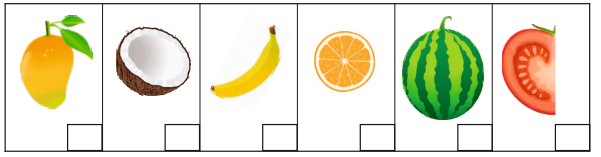Solution: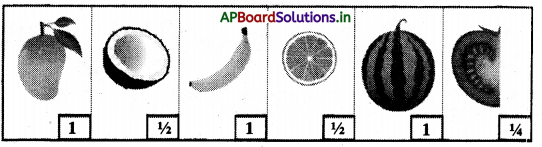Question 3.
Look at the following figures. A complete picture and its half are given. Find the other half from the list to make picture into a complete one.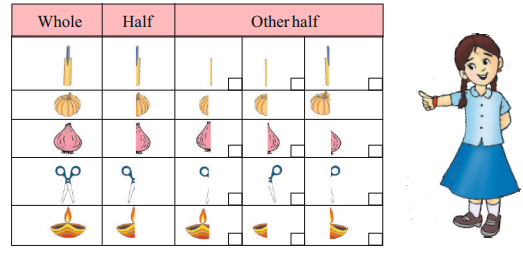Solution: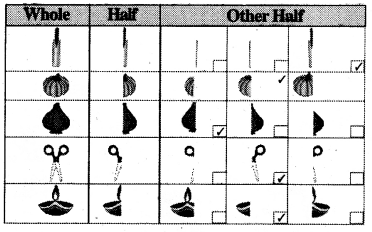Question 4.
Observe the following. Label the shaded part as half and quarter.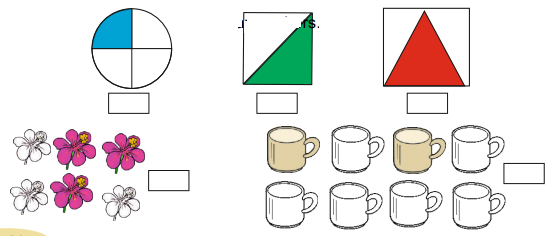Solution: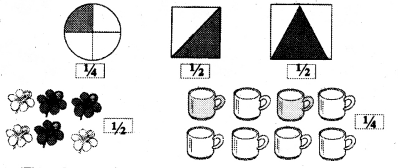Textbook Page No. 117

Do this

Question 1.
Shape one quarter of each of the following with a colour pencil. One Is done for you.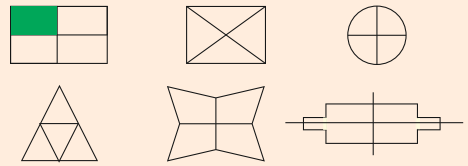Solution: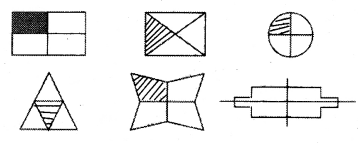Question 2.
Write “half’ or “quarter” in the boxes provided under each figures. One is done for you.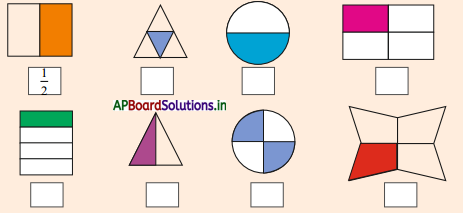Solution: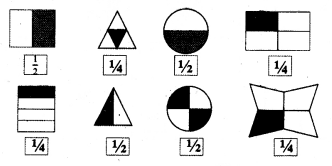Question 3.
Let us observe the following pictures and complete the table :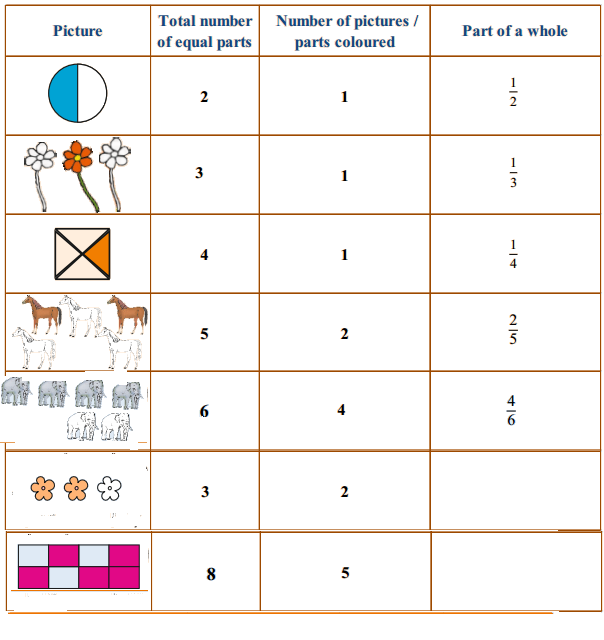Solution: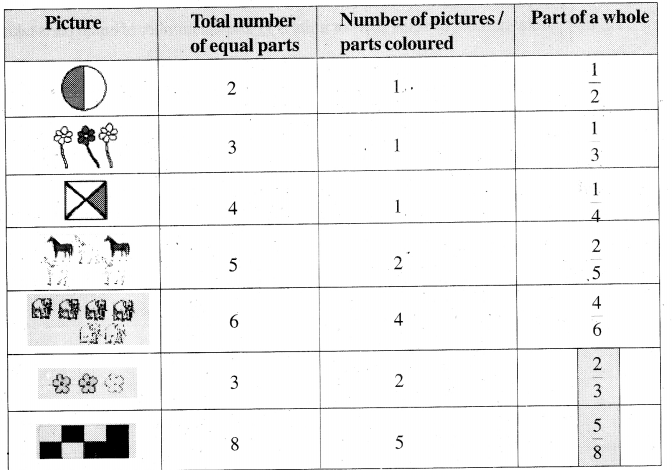Question 4.
Let us represent “part of a whole” or “fraction” with some more pictures.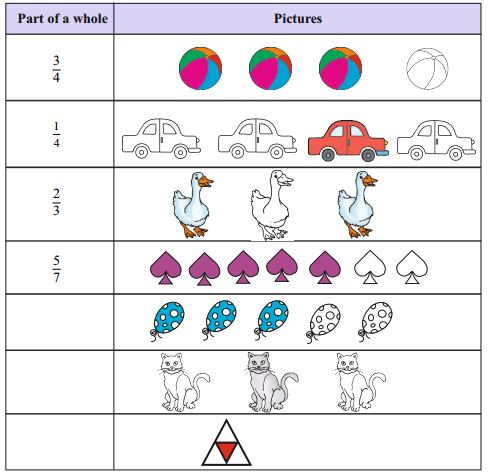Solution: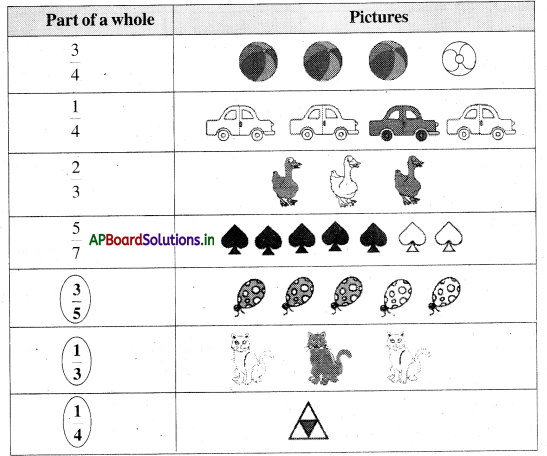Textbook Page No. 120

Do this

Question 1.
In $$\frac{3}{11}$$, total number of equal parts or units is ____________ and the number of equal parts taken is ____________.
Solution:
In $$\frac{3}{11}$$, total number of equal parts or units is            11               and the number of equal parts taken is             3                 .Question 2.
In $$\frac{3}{8}$$ total number of equal parts or units is __________ and the number of equal parts is taken is _________.
Solution:
In $$\frac{3}{8}$$ total number of equal parts or units is               8               and the number of equal parts is taken is                 3              .

Question 3.
In __________ the total number of equal parts are 8 and taken number of equal parts is 3.
Solution:
In            $$\frac{3}{8}$$              the total number of equal parts are 8 and taken number of equal parts is 3.

Question 4.
In $$\frac{2}{5}$$, total number of equal parts or units is __________; the shaded number of equal parts is __________ and the number of unshaded part is __________.
Solution:
In $$\frac{2}{5}$$, total number of equal parts or units is           5           ; the shaded number of equal parts is              2               and the number of unshaded part is             3            .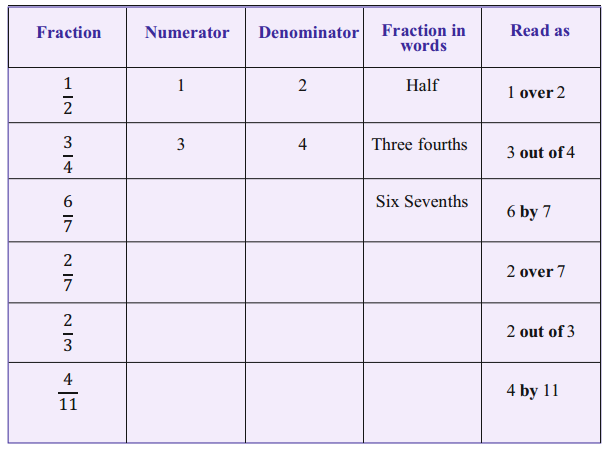Solution: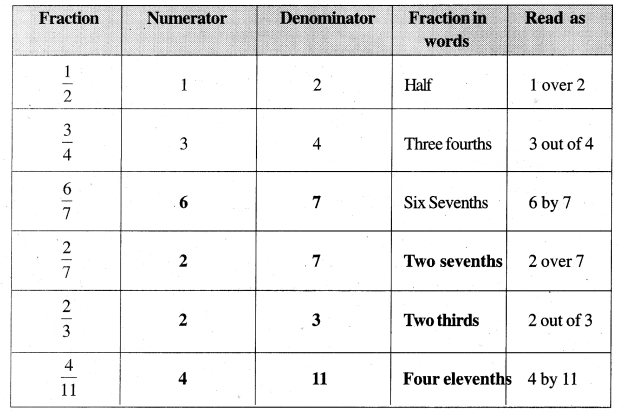Textbook Page No. 121

Think & Discuss

A student denoted the shade part as $$\frac{3}{5}$$. Is it correct ?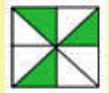Solution:
No, student is wrong. In the given picture, total number of equal parts is 8 and the number of equal parts shaded is 3.
∴ The shaded part as $$\frac{3}{8}$$.Textbook Page No. 123

Try this

Write some unit fractions as you like :
Solution:
a) $$\frac{1}{9}$$
b) $$\frac{1}{2}$$
c) $$\frac{1}{6}$$
d) $$\frac{1}{10}$$

Do this

Question 1.
Which is smaller $$\frac{1}{3}$$ or $$\frac{1}{5}$$ ?
Solution:
$$\frac{1}{5}$$ is smaller than $$\frac{1}{3}$$

Question 2.
Which is greater $$\frac{1}{12}$$ or $$\frac{1}{10}$$ ?
Solution:
$$\frac{1}{12}$$ smaller than $$\frac{1}{10}$$

Question 3.
Circle the smallest fractions:
$$\frac{1}{7}$$, $$\frac{1}{3}$$, $$\frac{1}{9}$$, $$\frac{1}{2}$$, $$\frac{1}{6}$$
Solution: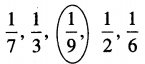Question 4.
Circle the greatest fractions:
$$\frac{1}{2}$$, $$\frac{1}{8}$$, $$\frac{1}{15}$$, $$\frac{1}{6}$$, $$\frac{1}{10}$$, $$\frac{1}{13}$$
Solution: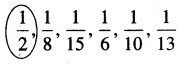Textbook Page No. 124

Try this

Arrange the following in ascending and descending order.
$$\frac{1}{9}$$, $$\frac{1}{2}$$, $$\frac{1}{6}$$, $$\frac{1}{8}$$, $$\frac{1}{4}$$, $$\frac{1}{15}$$, $$\frac{1}{3}$$
Solution:
Ascending order :
$$\frac{1}{15}$$ < $$\frac{1}{9}$$ < $$\frac{1}{8}$$ < $$\frac{1}{6}$$ < $$\frac{1}{3}$$ < $$\frac{1}{2}$$ Descending order : $$\frac{1}{2}$$ > $$\frac{1}{3}$$ > $$\frac{1}{6}$$ > $$\frac{1}{8}$$ > $$\frac{1}{9}$$ > $$\frac{1}{15}$$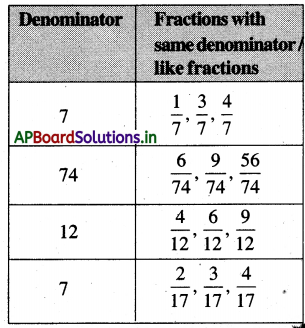Textbook Page No. 124

Do this

Question 1.
Circle the like fractions among the following.
$$\frac{2}{6}$$, $$\frac{3}{8}$$, $$\frac{1}{5}$$, $$\frac{4}{6}$$, $$\frac{2}{7}$$, $$\frac{5}{6}$$, $$\frac{3}{6}$$
Solution: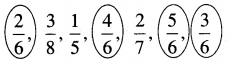Question 2.
Circle the other than like fractions among the following.
$$\frac{2}{5}$$, $$\frac{4}{7}$$, $$\frac{3}{5}$$, $$\frac{6}{9}$$, $$\frac{1}{5}$$, $$\frac{4}{5}$$, $$\frac{5}{8}$$
Solution: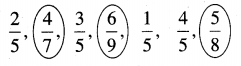Question 3.
Write like fractions of the following figures.
a)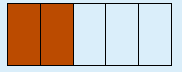Solution:
Fraction of shaded part $$\frac{2}{5}$$ like fraction
$$\frac{1}{5}$$, $$\frac{2}{5}$$, $$\frac{3}{5}$$, $$\frac{4}{5}$$
Fraction of unshaded part $$\frac{3}{5}$$

b)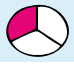Solution:
Fraction of shaded part $$\frac{1}{3}$$
Fraction of unshaded part $$\frac{2}{3}$$ like fractions $$\frac{1}{3}$$, $$\frac{2}{3}$$, $$\frac{3}{3}$$

Textbook Page No. 126

Do this

a) $$\frac{1}{7}$$ + $$\frac{2}{7}$$ = _____________
Solution:
$$\frac{1+2}{7}$$ = $$\frac{3}{7}$$

b) $$\frac{2}{5}$$ + $$\frac{2}{5}$$ = ______________
Solution:
$$\frac{2+2}{5}$$ = $$\frac{4}{5}$$c) $$\frac{3}{4}$$ + $$\frac{1}{4}$$ = _____________
Solution:
$$\frac{3+1}{4}$$ = $$\frac{4}{4}$$

d) $$\frac{2}{6}$$ + $$\frac{3}{6}$$ = _______________
Solution:
$$\frac{2+3}{6}$$ = $$\frac{5}{6}$$

e) $$\frac{4}{8}$$ + $$\frac{2}{8}$$ = ______________
Solution:
$$\frac{4+2}{8}$$ = $$\frac{6}{8}$$

f) $$\frac{5}{9}$$ + $$\frac{3}{9}$$ = ______________
Solution:
$$\frac{5+3}{9}$$ = $$\frac{8}{9}$$

Textbook Page No. 127

Do this

a) $$\frac{7}{12}$$ – $$\frac{5}{12}$$ = ____________
Solution:
$$\frac{7-5}{12}$$ = $$\frac{2}{12}$$

b) $$\frac{9}{10}$$ – $$\frac{3}{10}$$ = _____________
Solution:
$$\frac{9-3}{10}$$ = $$\frac{6}{10}$$c) $$\frac{18}{20}$$ – $$\frac{11}{20}$$ = _____________
Solution:
$$\frac{18-11}{20}$$ = $$\frac{7}{20}$$

Think and Discuss

a) $$\frac{1}{2}$$ + $$\frac{1}{2}$$ = _____________
Solution:
$$\frac{1+1}{2}$$ = $$\frac{2}{2}$$

b) $$\frac{3}{4}$$ + $$\frac{1}{4}$$ = ______________
Solution:
$$\frac{3+1}{4}$$ = $$\frac{4}{4}$$

c) $$\frac{1}{2}$$ – $$\frac{1}{2}$$ = _____________
Solution:
$$\frac{1-1}{2}$$ = $$\frac{0}{2}$$

Exercise – 9.1

1. If in each of the following, all the addends are the same, complete the circles.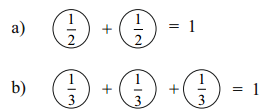c) _________ + __________ + __________ + __________ = 1
Solution:
$$\frac{1}{4}$$ + $$\frac{1}{4}$$ + $$\frac{1}{4}$$ + $$\frac{1}{4}$$ = 1d) __________ + __________ + __________ + __________ + __________ = 1
Solution:
$$\frac{1}{5}$$ + $$\frac{1}{5}$$ + $$\frac{1}{5}$$ + $$\frac{1}{5}$$ + $$\frac{1}{5}$$ = 1

e) __________ + __________ + __________ + __________ + __________ + __________ = 1
Solution:
$$\frac{1}{6}$$ + $$\frac{1}{6}$$ + $$\frac{1}{6}$$ + $$\frac{1}{6}$$ + $$\frac{1}{6}$$ + $$\frac{1}{6}$$= 1

f) __________ + __________ + __________ + __________ + __________ + __________ + __________ = 1
Solution:
$$\frac{1}{7}$$ + $$\frac{1}{7}$$ + $$\frac{1}{7}$$ + $$\frac{1}{7}$$ + $$\frac{1}{7}$$ + $$\frac{1}{7}$$ + $$\frac{1}{7}$$ = 1

2. Do the following additions. One is done for you.

a)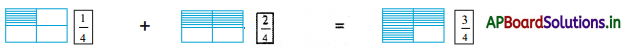b)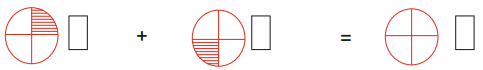Solution:
$$\frac{1}{4}$$ + $$\frac{1}{4}$$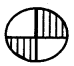= $$\frac{2}{4}$$

c)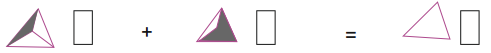Solution:
$$\frac{1}{3}$$ + $$\frac{2}{3}$$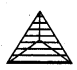= $$\frac{3}{3}$$d)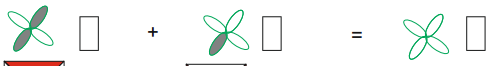Solution:
$$\frac{2}{4}$$ + $$\frac{1}{4}$$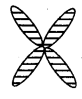= $$\frac{3}{4}$$

e)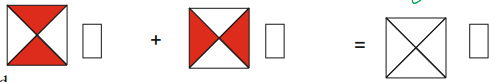Solution:
$$\frac{2}{4}$$ + $$\frac{2}{4}$$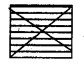= $$\frac{4}{4}$$

a) $$\frac{2}{7}$$ + $$\frac{1}{7}$$
Solution:
$$\frac{2+1}{7}$$ = $$\frac{3}{7}$$

b) $$\frac{5}{11}$$ + $$\frac{4}{11}$$
Solution:
$$\frac{5+4}{11}$$ = $$\frac{9}{11}$$c) $$\frac{2}{13}$$ + $$\frac{1}{13}$$ + $$\frac{5}{13}$$
Solution:
$$\frac{2+1+5}{13}$$ = $$\frac{8}{13}$$

Question 4.
Find the value of $$\frac{1}{3}$$ + $$\frac{5}{3}$$
Solution:
$$\frac{1}{3}$$ + $$\frac{5}{3}$$
= $$\frac{1+5}{3}$$
= $$\frac{6}{3}$$

5. Subtract the following.

a) $$\frac{7}{12}$$ – $$\frac{5}{12}$$
Solution:
$$\frac{7-5}{12}$$ = $$\frac{2}{12}$$

b) $$\frac{5}{9}$$ – $$\frac{1}{9}$$
Solution:
$$\frac{5-1}{9}$$ = $$\frac{4}{9}$$

c) $$\frac{8}{19}$$ – $$\frac{7}{19}$$
Solution:
$$\frac{8-7}{19}$$ = $$\frac{1}{19}$$Question 6.
Find $$\frac{4}{5}$$ – $$\frac{1}{5}$$
Solution:
$$\frac{4-1}{5}$$ = $$\frac{3}{5}$$

Textbook Page No. 129

Try this

a) Which is greater $$\frac{3}{7}$$ or $$\frac{5}{7}$$ ?
Solution:
$$\frac{3}{7}$$ is greater than $$\frac{5}{7}$$

b) Which is smaller $$\frac{2}{6}$$ or $$\frac{4}{6}$$ ?
Solution:
$$\frac{2}{6}$$ is greater than $$\frac{4}{6}$$

c) Arrange the following fraction in descending order.
$$\frac{1}{9}$$, $$\frac{7}{9}$$, $$\frac{3}{9}$$, $$\frac{5}{9}$$ and $$\frac{2}{9}$$
Solution:
Descending order :
$$\frac{7}{9}$$ > $$\frac{5}{9}$$ > $$\frac{3}{9}$$ > $$\frac{2}{9}$$ > $$\frac{1}{9}$$d) Arrange the following fractions in ascending order
$$\frac{7}{12}$$, $$\frac{3}{12}$$, $$\frac{5}{12}$$, $$\frac{11}{12}$$ and $$\frac{9}{12}$$
Solution:
Ascending order :
$$\frac{3}{12}$$ < $$\frac{5}{12}$$ < $$\frac{7}{12}$$ < $$\frac{9}{12}$$ < $$\frac{11}{12}$$

Exercise – 9.2

Question
1. Write the correct symbol > or < in the given boxes.
a) $$\frac{2}{8}$$ ___________ $$\frac{4}{8}$$
b) $$\frac{3}{10}$$ ___________ $$\frac{8}{10}$$
c) $$\frac{5}{7}$$ ___________ $$\frac{6}{7}$$
d) $$\frac{23}{25}$$ ___________ $$\frac{21}{25}$$
Solution:
a) $$\frac{2}{8}$$           <           $$\frac{4}{8}$$
b) $$\frac{3}{10}$$           <           $$\frac{8}{10}$$
c) $$\frac{5}{7}$$            <             $$\frac{6}{7}$$
d) $$\frac{23}{25}$$            >           $$\frac{21}{25}$$

2. Circle the smallest fraction.

a) $$\frac{7}{8}$$ , $$\frac{3}{8}$$, $$\frac{1}{8}$$, $$\frac{5}{8}$$, $$\frac{8}{8}$$
Solution: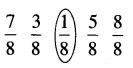b) $$\frac{7}{12}$$, $$\frac{3}{12}$$, $$\frac{5}{12}$$, $$\frac{11}{12}$$, $$\frac{9}{12}$$
Solution: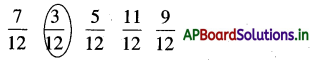3. Circle the greatest fraction.

a) $$\frac{3}{5}$$, $$\frac{4}{5}$$, $$\frac{1}{5}$$, $$\frac{2}{5}$$, $$\frac{5}{5}$$
Solution: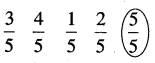b) $$\frac{17}{21}$$, $$\frac{5}{21}$$, $$\frac{20}{21}$$, $$\frac{10}{21}$$, $$\frac{2}{21}$$
Solution: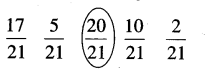4. Shade the following figure as per the given fractions and then compare.

a)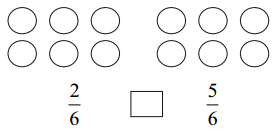Solution: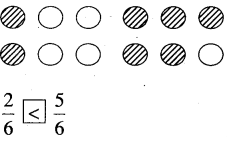b)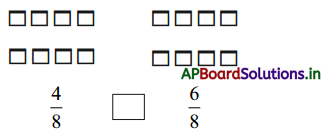Solution: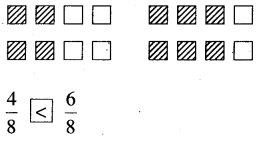5. Arrange the following in ascending order.

a) $$\frac{4}{9}$$, $$\frac{2}{9}$$, $$\frac{5}{9}$$, $$\frac{1}{9}$$, $$\frac{7}{9}$$
Solution:
Ascending order:
$$\frac{1}{9}$$ < $$\frac{2}{9}$$ < $$\frac{4}{9}$$ < $$\frac{5}{9}$$ < $$\frac{7}{9}$$b) $$\frac{4}{7}$$, $$\frac{2}{7}$$, $$\frac{3}{7}$$, $$\frac{5}{7}$$, $$\frac{1}{7}$$
Solution:
Ascending order:
$$\frac{1}{7}$$ < $$\frac{2}{7}$$ < $$\frac{3}{7}$$ < $$\frac{4}{7}$$ < $$\frac{5}{7}$$

6. Arrange the following in descending order.

a) $$\frac{14}{27}$$, $$\frac{4}{27}$$, $$\frac{21}{27}$$, $$\frac{15}{27}$$, $$\frac{2}{27}$$
Solution:
Descending order :
$$\frac{21}{27}$$ > $$\frac{15}{27}$$ > $$\frac{14}{27}$$ > $$\frac{4}{27}$$ > $$\frac{2}{27}$$

b) $$\frac{2}{7}$$, $$\frac{4}{7}$$, $$\frac{3}{7}$$, $$\frac{6}{7}$$, $$\frac{1}{7}$$, $$\frac{5}{7}$$
Solution:
Descending order :
$$\frac{6}{7}$$ > $$\frac{5}{7}$$ > $$\frac{4}{7}$$ > $$\frac{3}{7}$$ > $$\frac{2}{7}$$ > $$\frac{1}{7}$$

Exercise – 9.3

Question 1.
Arya adds $$\frac{4}{7}$$ litres of apple juice to $$\frac{2}{7}$$ litres of organge juice to make a fruit punch. How many litres of fruit punch does he have now?
Solution:
Quantity of apple juice = $$\frac{4}{7}$$ litres
Quantity of orange juice = $$\frac{2}{7}$$ litres
Quantity of fruit punch = $$\frac{4}{7}$$ + $$\frac{2}{7}$$
= $$\frac{4+2}{7}$$
= $$\frac{6}{7}$$ litresQuestion 2.
A piece of ribbon (of length) $$\frac{2}{9}$$ was cut from a ribbon (of length) $$\frac{5}{9}$$ units. What is the length of the remaining ribbon?
Solution:
Actual length of ribbon = $$\frac{5}{9}$$
Length of takenout piece = $$\frac{2}{9}$$
Length of remaining ribbon = $$\frac{5}{9}$$ – $$\frac{2}{9}$$
= $$\frac{3}{9}$$

Question 3.
If kishore ate $$\frac{9}{10}$$ of a pizza and Adi ate $$\frac{6}{10}$$ of another pizza of same size, then how much more pizza did kishore eat than Adi?
Solution:
Kishore ate pizza size = $$\frac{9}{10}$$
Adi ate pizza size = $$\frac{6}{10}$$
Difference = $$\frac{9}{10}$$ – $$\frac{6}{10}$$ = $$\frac{9-6}{10}$$ = $$\frac{3}{10}$$
Kishore ate $$\frac{3}{10}$$ than Adi.

Question 4.
A scooter tank has $$\frac{2}{3}$$ litres of petrol. Prasad rides around for some time and sees that the petrol level has come down to $$\frac{1}{3}$$ litres. How much petrol does he use for riding around ?
Solution:
Quantity of petrol in the scooter at first = $$\frac{2}{3}$$
Quantity of petrol in the after ride = $$\frac{1}{3}$$
Quantity of petrol he used = $$\frac{2}{3}$$ – $$\frac{1}{3}$$
= $$\frac{2-1}{3}$$ = $$\frac{1}{3}$$Question 5.
Krishna covered $$\frac{1}{5}$$th of his journey to school and suddenly he realizes that he had forgotten to bring his maths book. So, he walked back and returned to school in time. What is the extra distance he walked than usual ?
Solution:
Covered distance by Krishna at first = $$\frac{1}{5}$$
Again he covered distance = $$\frac{1}{5}$$
Total distance he covered = $$\frac{1}{5}$$ + $$\frac{1}{5}$$
= $$\frac{2}{5}$$

Multiple Choice Questions

Question 1.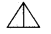each part is equal to
A) $$\frac{1}{2}$$
B) $$\frac{2}{1}$$
C) $$\frac{1}{4}$$
D) $$\frac{1}{3}$$
A) $$\frac{1}{2}$$

Question 2.
$$\frac{1}{2}$$ part of 12 eggs is equal to
A) 4
B) 3
C) 6
D) 13
C) 6Question 3.
5 equal parts taken from 8 equal parts is
A) $$\frac{8}{5}$$
B) $$\frac{8}{3}$$
C) $$\frac{3}{8}$$
D) $$\frac{5}{8}$$
D) $$\frac{5}{8}$$

Question 4.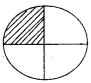shaded part is equal to
A) $$\frac{1}{4}$$
B) $$\frac{2}{4}$$
C) $$\frac{3}{4}$$
D) $$\frac{4}{4}$$
A) $$\frac{1}{4}$$

Question 5.
Fractional form of any one day month of June – 2020
A) $$\frac{2}{30}$$
B) $$\frac{3}{30}$$
C) $$\frac{4}{30}$$
D) $$\frac{1}{30}$$
D) $$\frac{1}{30}$$Question 6.
$$\frac{10}{10}$$ – $$\frac{2}{10}$$ = ?
A) $$\frac{5}{10}$$
B) $$\frac{8}{10}$$
C) $$\frac{10}{8}$$
D) $$\frac{1}{10}$$

Question 7.
$$\frac{5}{2}$$ = $$\frac{3}{2}$$ + ?
A) $$\frac{2}{5}$$
B) $$\frac{1}{2}$$
C) $$\frac{2}{2}$$
D) $$\frac{3}{2}$$
C) $$\frac{2}{2}$$

Question 8.
$$\frac{3}{4}$$ + $$\frac{1}{4}$$ = ?
A) $$\frac{4}{4}$$
B) $$\frac{4}{2}$$
C) $$\frac{2}{4}$$
D) $$\frac{0}{4}$$
A) $$\frac{4}{4}$$Question 9.
Among the following unit fraction
A) $$\frac{1}{9}$$, $$\frac{1}{2}$$, $$\frac{1}{3}$$, $$\frac{1}{6}$$
B) $$\frac{3}{10}$$, $$\frac{9}{10}$$, $$\frac{8}{10}$$
C) A and B
D) None
A) $$\frac{1}{9}$$, $$\frac{1}{2}$$, $$\frac{1}{3}$$, $$\frac{1}{6}$$
A) $$\frac{1}{9}$$, $$\frac{1}{2}$$, $$\frac{1}{3}$$, $$\frac{1}{6}$$
B) $$\frac{3}{10}$$, $$\frac{8}{10}$$, $$\frac{9}{10}$$, $$\frac{4}{10}$$
C) $$\frac{1}{2}$$, $$\frac{1}{3}$$, $$\frac{1}{4}$$, $$\frac{1}{5}$$
B) $$\frac{3}{10}$$, $$\frac{8}{10}$$, $$\frac{9}{10}$$, $$\frac{4}{10}$$